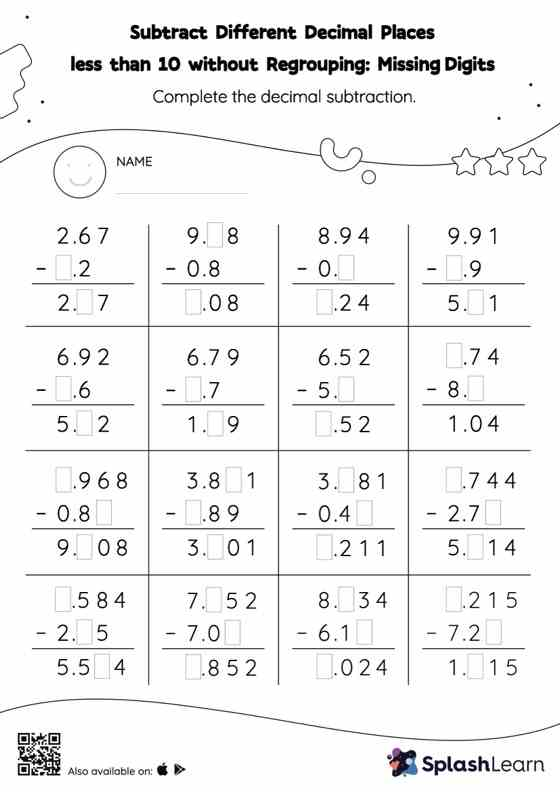# Subtract Different Decimal Places less than 10 without Regrouping: Missing Digits Worksheet

Home > Subtract Different Decimal Places less than 10 without Regrouping: Missing DigitsThis worksheet gives students an opportunity to apply the concepts of subtraction to subtract different decimal places less than 10 without regrouping. In each problem, the numbers are laid out in the vertical format. In this format, pair of digits in each successive place are tackled one by one. This helps students follow a structured approach.